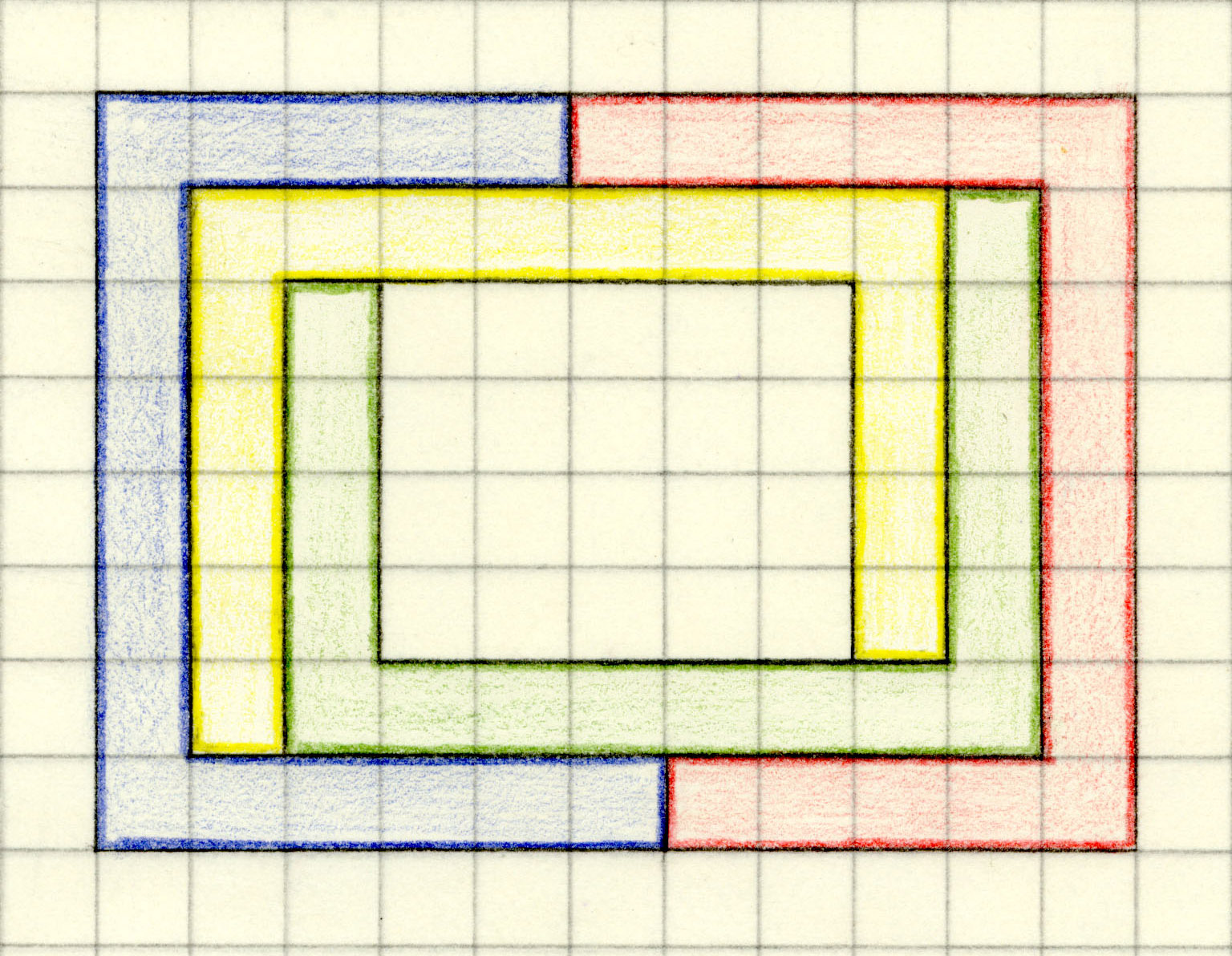17. Can two tiles surround the others?

This is no problem for tetrads with holes.Here I present the first tetrads with no holes where two tiles completely surround the others.
These also are the first centrally symmetric tetrads with no hole (as far as I know).
This tetrad with 20-gon tiles was constructed on February 22th, 2020.
It is possible to tile the plane with such a 20-gon.The construction of the new tiles is also possible with individual curves.
This one was found on February 23th, 2020. 24 tiles can be arranged in order to form a blossom.
These component regions cannot tile the plane.What was the initial idea for the new tiles?

Andrejs Cibulis made me aware of an article written by Casey Mann in 2002 .
There Casey Mann showed that three congruent modified Voderberg tiles , can be arranged in a way that two tiles completely surround the third tile. The modification was made rather complicated by 'hooks' and 'catches'. It was also discussed how to two tiles can surround n copies of the tiles. But with the presented modification in the case n = 2 two pairs of tiles would only touch in a common vertex and not in a piece of their boundaries.
By cutting off small isosceles triangles from the corners with acute angles, I could improve Mann's modifications and construct the tetrad.Details of cornersImproved construction method for the new tiles

By a variation of the Voderberg nonagon construction we can obtain polygons directly without Mann's method. In Voderberg tiles the center of rotation is a vertex of the nonagon. For the new tiles the center of the rotation is outside the polygon. The smallest possible number of vertices is 16.
It is possible to tile the plane with such a 16-gon. The outline of the tetrad is a 14-gon.
The common parts of the edges are rather short and the vertices are difficult to see, unless they are additionally marked. Therefore I prefer the 20-gon at the top of this page.

Coordinates of the generating path.Construction of the extended Voderberg tiles

Choose a unit length u, a radius r (for example r = u) and an angle δ (for example 15°).
Construct the points of the graphic below. Create a path from vertex S to T. This path has to be simple (no intersections) and you have to avoid intersection when the necessary transformations were made. A straight line from S to T is not suitable.
A straight line from V to W works, but also an individual path is possible. An individual path from T to U has to be rotated around Q with angle δ in order to obtain the path from U to V.
If the tile shall tile the plane you need a point symmetric path from V to W and a straight line from U to V.The red path from S to W defines the tile.

Rotate the red path counterclockwise around R with angle δ, in order to obtain the green path from O to Z.
Translate the path from S to U according to the vector from S to S', in order to obtain the blue path from S' to W.
Reflect the red, green and blue paths at the Origin O. The light green and yellow tiles are finished.
Rotate the light green tile clockwise around the point R with angle δ, in order to obtain the light red tile.
Rotate the yellow tile clockwise around the point R' with angle δ, in order to obtain the light blue tile.Very strange tiles are possible

A tetrad with congruent strange tiles24 of these tiles can form a 'fire wheel'Tiling the plane

As example the 16-gon-tile is used here.A wheel made of the 16-gon tiles with the colors of the U.S. Flag (30 tiles because δ = 12°)